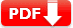# ACT Math Practice Test 1 (60 Question Full Test OR Review Question Solution)

ACT Math Practice Test 1 (60 Question Full Test OR Download PDF): Students can either download free printable ACT Math practice test question answers or participate in the full-length online test with 60 minutes time limit. You can also prepare your math skill by reviewing the questions with solution.

Test Name ACT Test Prep Free ACT Practice Test algebra, geometry, and trigonometry Sample Multiple Choice either join the free full-length test or review question and solution 60 60 minute (1 hour) YES YES YES

## ACT Math Practice Test Online Quiz(60 Question1 hr. Full Test)
To Stat the Quiz – Click on “Start Quiz Button” as shown below

FREE ACT Full Math Practice Test – 1

## ACT Math Practice Test 1 (Review & Solution)

For each problem, choose the correct answer. You are allowed to use a calculator on this test for any problems you choose. Unless the problem states otherwise, you should assume that figures are not drawn to scale. For this test, all geometric figures lie in a plane, the word line refers to a straight line, and the word average refers to the arithmetic mean.

1. The expression a[b – (c – d)] is equivalent to

• A). ab – ac – ad
• B). ab + ac – ad
• C). ab – c – d
• D). ab – ac + ad
• E). ab – c + d

Solution: First distribute the negative sign outside the parentheses, which changes the d to a positive value. Then distribute the a to all three terms.

Q2. To date, a student has four test scores of 81, 87, 91, and 86. What must he receive on his fifth test to achieve an average of 88 for the five tests?

• A). 65
• B). 75
• C). 85
• D). 90
• E). 95

Solution: To average 88 for five tests, one would need an aggregate score of 440. The first four tests total 345, meaning that a 95 would be necessary on the final test.

Q3. What two distinct integers should be placed in the blank spaces so that the difference between the four integers is the same? 23, ____, ____, 62

• A). 36, 49
• B). 27, 43
• C). 33, 39
• D). 29, 49
• E). 26, 39

Solution: The separation between 23 and 62 is 39, and adding two numbers in between will create three gaps, so each gap must be a space of 13.

Q4. Sasha ran 2 \frac13  miles on Monday,  3 \frac34  miles on Tuesday, and 5 \frac23 miles on Wednesday. How many miles in total did she run in those three days?

• A). 10 \frac13
• B). 10 \frac49
• C).  11 \frac59
• D). 11 \frac34
• E). 12 \frac19

Solution: There is no need to find a common denominator; simply add the distances from Monday and Wednesday (exactly 8), and then add Tuesday’s distance to that total..

Q5. What is the value of the expression h (h + 1)2 for h = 3?

• A). 14
• B). 32
• C). 48
• D). 97
• E). 144

Solution: The proper order of operations is to add the values inside the parentheses, square that value, and then multiply it by 3.

Q6. Company X sells 80 markers for $49.60, and Company Y sells 125 of the same markers for$72.50. Which company offers a better price per marker, and how much less is its price?

• A). Company X, 4 cents
• B). Company X, 2 cents
• C). Company Y, 4 cents
• D). Company Y, 58 cents
• E).The costs are the same

Solution: Simple division shows that Company X sells its pens for 62 cents each, and Company Y sells them for 58 cents each (4 cents less)

Q7. A rectangular lot is entirely enclosed by a fence. If the lot measures 225 feet long and 165 feet wide, what is the length, in yards, of the fence?

• A). 260
• B). 360
• C). 960
• D). 1160
• E). 1260

Solution: The perimeter of a rectangle is found by adding two widths and two lengths. Divide by 3 to convert length to yards.

Q8. A ladder is 16 feet long and reaches 10 feet up a wall. Approximately how many feet from the bottom of the wall is the base of the ladder?

• A). 5.5
• B). 8.5
• C). 9
• D). 10.9
• E). 12.5

Solution: Use the Pythagorean theorem to find the missing value, in this case the short leg of the triangle.

Q9. If 7x – 12 = 11x + 24, then x =

• A).  –1
• B). –3
• C). –5
• D). –6
• E). –9

Solution: Use basic algebra and first subtract 7x from both sides, then subtract 24 from both sides.

Q10. Mr Johnson teaches fifth grade at Littleton Elementary School and earns $21,850 for teaching a 190-day school year. He is going on a five-day vacation, and his substitute will be paid$90 per day. How much money will Littleton Elementary School save while Mr Johnson is away?

• A). $125 • B).$155
• C). $325 • D).$1125
• E). $2125 Show Answers Answer: A Solution: Simple division shows that Mr Johnson earns$115 per day, or $25 more than his substitute, which will be$125 saved over a five-day period.

Q11. If x is a real number so that x3 = 125, then 2(x2 + x) = ?

• A). 15
• B). 30
• C). 60
• D). 120
• E). 160

Solution: First solve for x, which must equal 5, and then plug it into the equation. Following proper order of operations, first square 5, then add it to 5, and then multiply the sum by 2.

Q12. If a marble is chosen at random from a bag that contains 16 blue marbles, 12 red marbles, 6 green marbles, and 10 white marbles, what is the probability that the marble chosen will NOT be blue?

• A). 7/11
• B). 9/11
• C). 16/11
• D). 1/11
• E). 17/11

Solution: Probability is found by dividing the desired outcome by all possible outcomes. In this case, the desired outcome is “not blue,” which has 28 possibilities, and the total number of outcomes (marbles) is 44. Then reduce the fraction.

Q13. For all values of x, the quantity (5x + 2)2

• A). 10x2 + 4
• B). 25x2 + 4
• C). 25x2 + 10x + 4
• D). 10x2 + 10x + 4
• E). 25x2 + 20x + 4

Solution: Proper technique for squaring the expression—first times first, outside times outside, inside times inside, and last times last (FOIL)—yields the correct answer.

Q14. On the number line of all real numbers, what is the midpoint of –12 and 20?

• A). 1
• B). -2
• C). 4
• D). 6
• E). 10

Solution: The two values are separated by 32, which means the midpoint must be 16 from each one..

Use the following information to answer questions 15 through 17. The chart shows the current enrollment in classes at St. Middle School.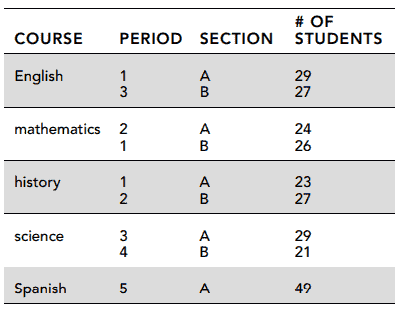Q15. What is the average number of students taking a class during period 1?

• A). 22
• B). 23
• C). 27
• D). 26
• E). 28

Solution: The chart indicates there are three-period 1 classes, with enrollments of 23, 26, and 29, and thus an average of 26.

Q16. During which period do the fewest students take class?

• A). 2
• B). 4
• C). 1
• D). 3
• E). 5

Solution: Period 4 has only one class, with an enrollment of 21

Q17. The school owns 52 chairs, but 6 are broken. For which, period(s) will there not be enough chairs to seat all of the students in classes?

• A). period 1 only
• B). period 4 only
• C). periods 1 and 4
• D). all but period 4
• E). all of the periods

Solution: Period 1 has 78 students, period 2 has 51 students, period 3 has 56 students, and period 5 has 49 students. Only period 4 has fewer than 46 students.

Q18. The formula for the volume V of a sphere with a radius of r is V = 3/4 π r3. If a spherical ball has a radius of 3 (1/4) inch, what is its volume to the nearest cubic inch?

• A). 9
• B). 13
• C). 21
• D). 83
• E). 143

Solution: First plug in the value for the radius, then cube that value, and then multiply by both π (3.14) and 4/3.

Q19. Celia has five blouses, four pairs of pants, three scarves, and three hats. If every outfit must include one blouse, one pair of pants, one scarf, and one hat, how many different outfits can Celia create?

• A). 15
• B). 30
• C). 90
• D). 180
• E). 360

Solution: To find the number of combinations created by a series of options, in this case types of clothing, multiply the numbers. So the options for Celia are 5 × 4 × 3 × 3, or 180.

Q20. Wool socks cost $9.60 for a package of three pairs of socks. If you buy three packages, you receive one free pair of wool socks. What would be the average cost of a pair of socks when you buy three packages? • A).$2.60
• B). $2.80 • C).$2.88
• D). $3.08 • E).$3.20
Solution: The total cost of the three packages would be $28.80, and the total number of pairs of socks would be 9 + 1, or 10. Then use simple division to find the cost per pair of socks. Q21. For the system of linear equations shown, which of the following would describe the graph of this system in a standard coordinate plane? 2y = –4x + 7 and 2y = 4x – 7 • A). a single line with a positive slope • B). a single line with a negative slope • C). two parallel lines with positive slopes • D). two parallel lines with negative slopes • E). two distinct intersecting lines Show Answers Answer: E Solution: The two equations have different slopes, –2 and 2, and therefore are two distinct lines that intersect in one point. Q22. Which of the following is a solution to the quadratic equation x2 – 24x = 0? • A). 24 • B). -21 • C). -36 • D). 23 • E). 2 Show Answers Answer: A Solution: By factoring out an x, you get x(x – 24) = 0, and plugging 24 in for x yields an answer of 0. Q23. At Mount Pleasant Ski Resort, 300,000 tons of snow are required to cover 1.5 acres of ski slope. How much snow would be required to cover 6.5 acres? • A). 1,333,000 tons • B). 1,300,000 tons • C). 1,700,000 tons • D). 1,255,000 tons • E). 900,000 tons Show Answers Answer: B Solution: Set up a proportion of (300,000/1.5) = (x/6.50) cross multiply, and solve for x. Q24. In the right triangle Δ ABC shown, tangent B =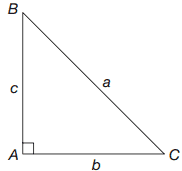• A). b/c • B). a/b • C). c/a • D). a/c • E). b/a Show Answers Answer: A Solution: The tangent of an angle is found by dividing the opposite side by the adjacent side. Q25. A rectangle measures 32 yards by 46 yards. What is the approximate measurement, in feet, of the diagonal of this rectangle? • A). 16 • B). 68 • C). 168 • D). 268 • E). 368 Show Answers Answer: C Solution: Using the Pythagorean theorem, the diagonal is the square root of 322 + 462, or 56 yards. Multiply by 3 to convert to feet. Q26. For what values of x will the equation (x + a)(x – a) = 0 be true? • A). a and x • B). –a and –x • C). x and 0 • D). –a and a • E). none of the above Show Answers Answer: D Solution: Set each of the two factors, (x + a) and (x – a), equal to 0, and solve for x Q27. On the number line shown, an object moves from A to B, from B to C, from C to D, and finally from D to E. What is the total distance the object moves?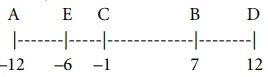• A). 12 • B). 18 • C). 36 • D). 47 • E). 58 Show Answers Answer: E Solution: Find the difference between the coordinates in each of the moves, and add the four differences together. Q28. What number can you add to the numerator and denominator of \frac{8}{13} to get 6? • A). -4 • B). -12 • C). -14 • D). 4 • E). 13 Show Answers Answer: C Solution: Adding –14 to both the top and the bottom of the fraction creates a new fraction of \frac{-6}{-1} or +6. Q29. For what value of x is the equation logx 64 = 3? • A). 1 • B). 2 • C). 4 • D). 8 • E). 16 Show Answers Answer: C Solution: logx64 = 3 means x3 = 64, so x = 4. Q30. In circle C, shown here, a chord 32 inches long is 10 inches from the center of the circle. What is the radius of the circle, to the nearest tenth of an inch?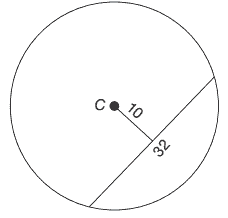• A). 18.9 • B). 16.7 • C). 21.9 • D). 19.3 • E). 14.9 Show Answers Answer: A Solution: The distance from the center meets the chord at a right angle at its midpoint. You then use the Pythagorean theorem to solve for the radius, which is 18.86, or 18.9 to the nearest tenth. Q31. The formula for the length of a spring is given by the equation L= \frac23 F + 0.03 where F is the applied force, in newtons. What is the force, in newtons, if the length of the spring is 0.83 meters? • A). 0.5 • B). 1.2 • C). 1.8 • D). 2.2 • E). 0.3 Show Answers Answer: B Solution: Simply plug 0.83 in for L, and then solve for F by first subtracting 0.03 from both sides and then dividing both sides by 2/3. Q32. If y = x + 2, then (x – y)5 = • A). –32 • B). –16 • C). –62 • D). –39 • E). 123 Show Answers Answer: A Solution: Replace y in the second equation with (x + 2), and then subtract that quantity from x. The result is –2, which is then raised to the fifth power. Q33. A mall has the shape and dimensions, in blocks, indicated in the diagram. There is a statue located halfway in between points E and B. What is the location of the statue relative to point C?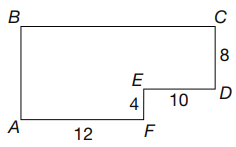• A). 16 blocks west and 4 blocks south • B). 17 blocks west and 5 blocks south • C). 14 blocks west and 8 blocks south • D). 18 blocks west and 5 blocks north • E). 6 blocks east and 4 blocks north Show Answers Answer: A Solution: The distance between E and B (east to west) along BC is 12, so half of that is 6. Add that to the distance between E and C (west to east) along with ED, which is 10, and the statue is 16 west of C. The distance between B and E (north to south) along BA is 8. Half of that is 4, so the statue is 4 south of C. Q34. A circle C is inscribed in a square as shown. If the area of the square is 256 units square, what is the radius of the circle?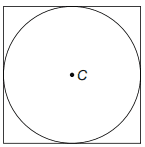• A). 2 units • B). 4 units • C). 8 units • D). 16 units • E). 32 units Show Answers Answer: C Solution: If the area of the square is 256, then the length of one side is the square root of 256, or 16, and the radius of the circle is half of the length of the side of the square. Q35. In the standard coordinate plane, what is the y-coordinate of the point of intersection of the two lines y = 4x – 6 and y = 2x + 2? • A). 4 • B). 6 • C). 10 • D). 18 • E). 30 Show Answers Answer: C Solution: By setting the two equations equal to each other and solving for x, you find x = 4. Then plug that value in for x in either equation and solve for y. Q36. The ratio of three sides of a triangle is 16:19:28. If the length of the short side of a similar triangle is 20, what is the length of the longest side of the similar triangle? • A). 29 • B). 31 • C). 32 • D). 33 • E). 35 Show Answers Answer: E Solution: Set up a proportion, where 16/20 = 28/x. Cross multiply, so 16x = 20 × 28, and divide both sides by 16. Q37. Which of the following inequalities describes the solution set for the inequality 25 – 6x ≥ 9? • A). x ≥ \frac83 • B). x ≥ - \frac83 • C). x ≤ - \frac83 • D). x ≤ \frac83 • E). x ≥ \frac23 Show Answers Answer: D Solution: Using basic algebra to solve, subtract 25 from both sides, divide both sides by –6, and reverse the inequality sign when dividing by a negative number. Q38. The solution set of \sqrt{x-1} > 7 is the set of all real numbers where • A). x > 6 • B). x > 9 • C). x > 50 • D). x > 62 • E). x > 72 Show Answers Answer: C Solution: Since the square root of 49 is 7, the value in the square root sign must be greater than 49, and x must be greater than 50. Q39. In the standard coordinate plane, what is the distance between the points (6,–2) and (–4,8)? • A). √18 • B). 10√2 • C). 10√6 • D). 10√9 • E). 10√7 Show Answers Answer: C Solution: Using the distance formula, find the square of the difference in the y coordinates (–2 minus 8, or –10, which squared is 100) and the square of the difference of the x coordinates (6 minus –4, or 10, which squared is 100), and add them (200). Then take the square root of 200.. Q40. In the figure, points A, B, C, and D are collinear. Angles A, E, F, and D are as marked. What is the measure of angle G?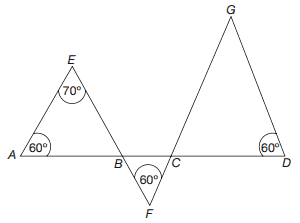• A). 50° • B). 70° • C). 80° • D). 90° • E). 110° Show Answers Answer: A Solution: Since the angles of a triangle add up to 180°, ∠ABE must equal 50°. Therefore, by vertical angles, ∠CBF is also 50°. Repeat this process to solve for ∠BCF and ∠DCG, and then for ∠G. Q41. Light travels at approximately 186,000 miles per second. At that rate, approximately how many miles will it travel in 3 hours? • A). 1.6 × 105 • B). 1.6 × 108 • C). 2.0 × 108 • D). 2.0 × 109 • E). 2.2 × 109 Show Answers Answer: C Solution: To solve, multiply 186,000 by 60 to find the distance per minute, then by 180 to find the distance in 3 hours. Convert the answer to scientific notation.. Q42. The ratio of the diameters of two circles is 6:10. What is the ratio of their areas? • A). 3:5 • B). 3:25 • C). 6:5 • D). 6:25 • E). 9:25 Show Answers Answer: E Solution: If the ratio of the diameter of the two circles is 6:10, then the radius is 3:5. The formula for the area of a circle is Πr2, so the ratio of the areas is 9Π:25Π, or 9:25. Q43. Hexagons, as pictured here, have nine diagonals. How many diagonals does the octagon have?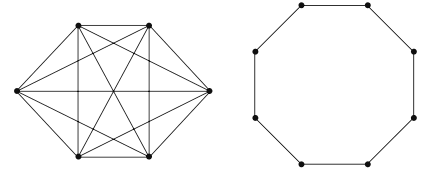• A). 10 • B). 16 • C). 20 • D). 24 • E). 28 Show Answers Answer: C Solution: Working systematically, connect each vertex to every other, not including the sides of the octagon. Q44. If 80% of a number is 260, what is 116% of the same number? • A). 311 • B). 317 • C). 349 • D). 377 • E). 397 Show Answers Answer: D Solution: To start, let 0.80x = 260. Then multiply the solution by 116%, or 1.16, to find the answer. Q45. Of the 504 girls in Radcliffe Day School, \frac38 are left-handed and \frac23 of those have brown hair. How many brown-haired, left-handed girls attend Radcliffe Day School? • A). 94 • B). 126 • C). 136 • D). 156 • E). 166 Show Answers Answer: B Solution: Multiply 504 times \frac38 (0.375), or divide 504 by 8 and then multiply that answer by 3. Then take this answer and multiply by \frac23 , or divide it by 3 and then multiply by 2. Q46. As shown here, Xs are arranged in a triangular pattern by adding two Xs to each additional row. If n = number of rows, which of the following properly describes the total number of Xs in the triangular pattern for any value of n? X X X X X X X X X X X X X X X X X X X X X X X X X X • A). n + 2 • B). n – 3 • C). 2n – 1 • D). 2n + 2 • E). n2 Show Answers Answer: E Solution: Try each answer. The first row contains one x, the first two rows contain four x’s, the first three rows contain nine x’s, the first four rows contain 16 x’s, so the rule is that n rows contain n2 x’s. Q47. In the right triangle pictured, sin(C) × tan(B) =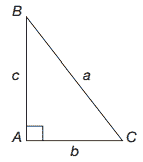• A). a2 • B). a/b • C). b/a • D). 1 • E). b2 Show Answers Answer: C Solution: sin(C) = \frac{opp}{hyp} or \frac{c}{a,} and tan(B) = \frac{opp}{adj} or \frac{b}{c} Then multiply \frac{c}{a} times \frac{b}{c} Q48. Alice is about to cover a patch of her backyard with decorative bricks that measure 2 inches by 6 inches. The area she wishes to cover measures 6 feet by 12 feet. What is the minimum number of bricks she must use to cover such an area? • A). 720 • B). 864 • C). 995 • D). 1024 • E). 1864 Show Answers Answer: B Solution: First, convert the dimensions of space to be covered to inches, and then find the area (10,368 inches2). Then find the area of each brick (12 inches2), and divide into the total area. Check to confirm that the bricks will fit evenly into space, which they will since both of the dimensions (in inches) are divisible by both 2 and 6. Q49. If the imaginary number i2 = –1, then the complex number operation of \frac{1}{i-1} \frac{i+1}{i+1} =? • A). i – 1 • B). i + 1 • C). 1 – i • D). (i + 1) / 2 • E). (i + 1) / -2 Show Answers Answer: E Solution: Multiply the numerator times the numerator and the denominator times the denominator. If you properly FOIL the denominator, the answer is \frac{i + 1} {i^2 - 1} Then convert i2 to –1. Q50. In the standard coordinate plane, a circle is tangent to the y-axis at 5 and tangent to the x-axis at –5. What is the equation of the circle? • A). x2 + y2 = 5 • B). x2 + y2 = 25 • C). (x + 5)2 + (y – 5)2 = 25 • D). (x + 5)2 + (y + 5)2 = 25 • E). (x – 5)2 + (y + 5)2 = 25 Show Answers Answer: C Solution: Since the circle is tangent to the indicated points, its center must be at (–5,5), and it must have a radius of 5. These are the values (h,k) and r in the equation of a circle. Then plug these values into the equation for a circle, which is (x – h)2 + (y – k)2 = r2. Q51. If the ratio of x:y is 4:5, and the ratio of y:z is 8:9, what is the ratio of x:z? • A). 8:10 • B). 16:15 • C). 32:45 • D). 36: 40 • E). 55:10 Show Answers Answer: C Solution: Convert the y values to a common value of 40 by multiplying the first ratio by 8 and the second ratio by 5. The x value becomes 32, and the z value becomes 45. Q52. There are 420 people living in a small town. The 190 men have an average age of 42, and the women have an average age of 37. What is the average age, to the nearest year, of the residents of the town? • A). 39 • B). 40 • C). 45 • D). 49 • E). 51 Show Answers Answer: C Solution: An average is found by dividing the total amount (in this case, years) by the total number (in this case, people). Multiply the number of men by their average age, the number of women (found by subtracting 190 from 420) by their average age, add these two values together and divide by 420. Q53. In the graph, the x-axis is intersected by the graph at π/2, 3π/2, 5π/2, 7π/2, and so on. The curve over anyone interval is a repetition of the other intervals. What is the period of the function?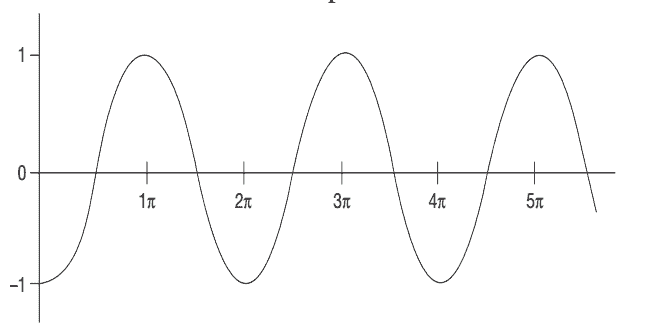• A). π/2 • B). π • C). 2π • D). 3π • E). 3π/2 Show Answers Answer: C Solution: The period is found by measuring the distance the graph takes to complete one full curve from y = –1 to y = –1. This full curve takes place over a distance of 2π. Q54. Two triangular prisms are similar. The smaller one has a triangular face with an altitude of 10, and the larger one has a triangular face with an altitude of 15. If the volume of the smaller prism is 4,000, what is the volume of the larger prism? • A). 9,500 • B). 11,500 • C). 13,500 • D). 16,500 • E). 17,500 Show Answers Answer: C Solution: The linear relationship of the corresponding parts of the similar prisms is 10:15, or 2:3. Therefore, the cubic relationship of the prisms must be (2:3)3, or 8:27. Set up a proportion of 8:27 = 4,000:x, and solve. Q55. If (x – 3) is a factor of the equation 3×2 – 11x + n, what is the value of n? • A). –6 • B). 6 • C). 10 • D). 24 • E). 32 Show Answers Answer: B Solution: If ( x – 3) is a factor of 3x2 – 11x + n, then (3x – a) must be a factor as well, where (–3)(–a) = n, and (–3)(3) + (–a)(1) = –11. Solve for a in the second equation, and a = –2, and then plug that value into the first equation to solve for n. Q56. If f(x) = 2x2 + 2, then f(x + k) = • A). 2x2 + 4kx + 2 • B). 2x2 + 2k + 2 • C). 2x2 + 2k2 + 2 • D). 4x2 + 2k2 + 2 • E). 2x2 + 4kx + 2k2 + 2 Show Answers Answer: E Solution: Plug (x + k) into the function 2x2 + 2 in place of x, then square (x + k), distribute the 2, and then add 2. Q57. If sin a =- \frac45 and π < a < \frac{3π}{2} then then cos a = • A). -(3/5) • B). 3/5 • C). 4/5 • D). -4/5 • E). 3/4 Show Answers Answer: A Solution: Sin is equal to \frac{opp}{hyp} or \frac{y}{hyp} therefore the y-value is –4, and the hypotenuse is 5. This indicates a 3–4–5 right triangle, so the missing side is 3. Since the angle must be less than 3π/2 , it must be located in the third quadrant of a standard (x,y) coordinate plane, and x must therefore be –3. Cos is \frac{adj}{hyp} or \frac{x}{hyp} Q58. Which of the following graphs would be the correct graph of the equation y = \frac{3x^2+3x}{x}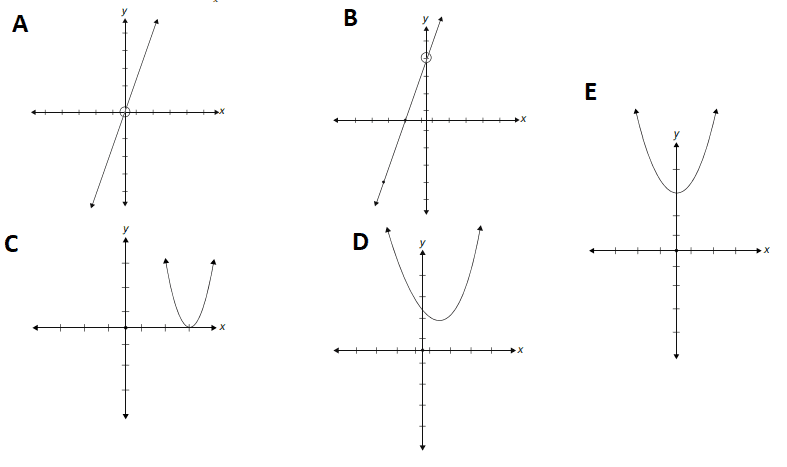Show Answers Answer: B Solution: While this seems too complicated to be a linear graph, it is. Factor out an x in the numerator, and you are left with 3x + 3; the numerator and denominator x’s cancel out. However, the graph is undefined at x = 0, so there is a hole in the graph at that value. Q59. Given the pair of equations A = 6C + 4 and B = –3C – 5, express the value of A in terms of B. • A). –2B – 6 • B). 2B – 6 • C). –2B + 6 • D). 3B – 1 • E). 2B + 6 Show Answers Answer: A Solution: First, use algebra to solve for C in terms of A in the first equation. Then replace C in the second equation with the value found in the first equation. Now, again use basic algebra to solve for A in the second equation. Q60. A guitar was marked to be sold in a store for$120 and then marked down 15%. A month later it was marked down an additional 5%. If the same guitar was available online for $100, what percent could be saved by buying it at the store after the second markdown? • A). 0.31% • B). 0.4% • C). 3.1% • D). 4.0% • E). 23.1% Show Answers Answer: C Solution: First, find 15% of$120 and subtract that from $120, leaving$102. Next, subtract 5% of $102 from$102, leaving $96.90. Now find the difference between this number and$100, and determine what percent of \$100 it is.
 Document Type Download Link Free Editable Doc File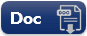Free Printable PDF File